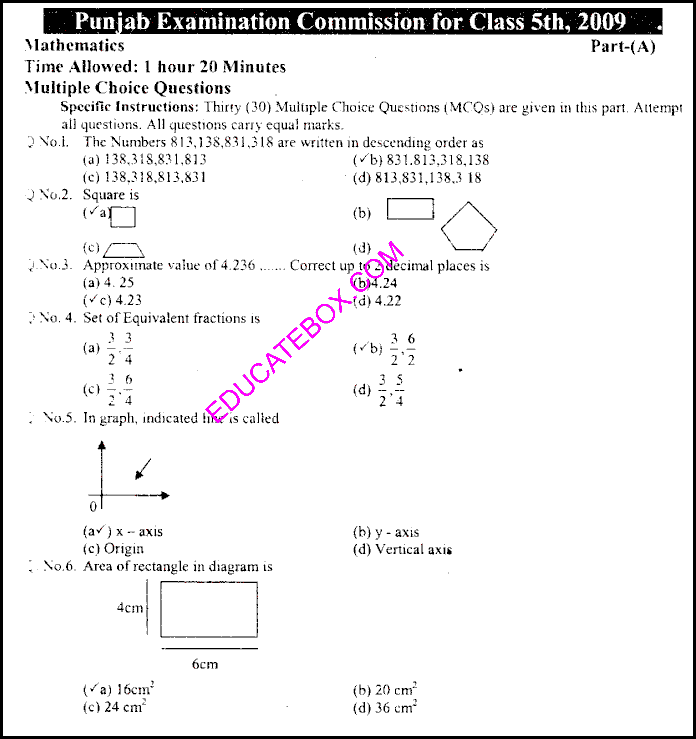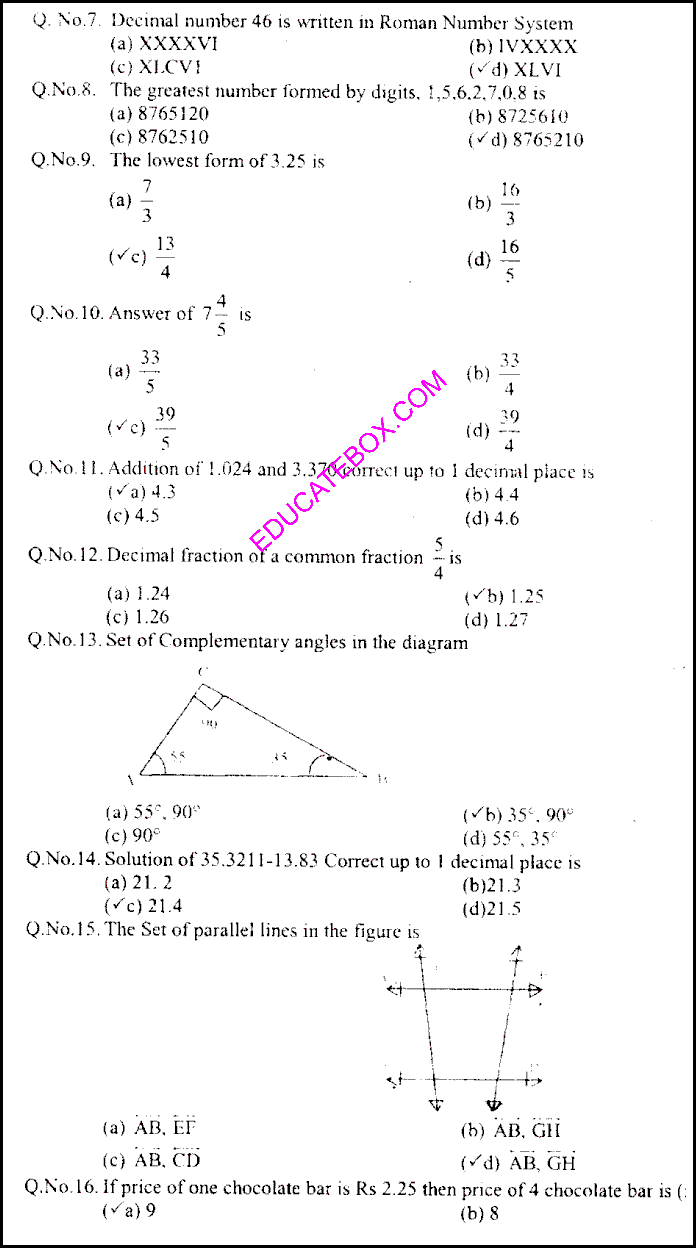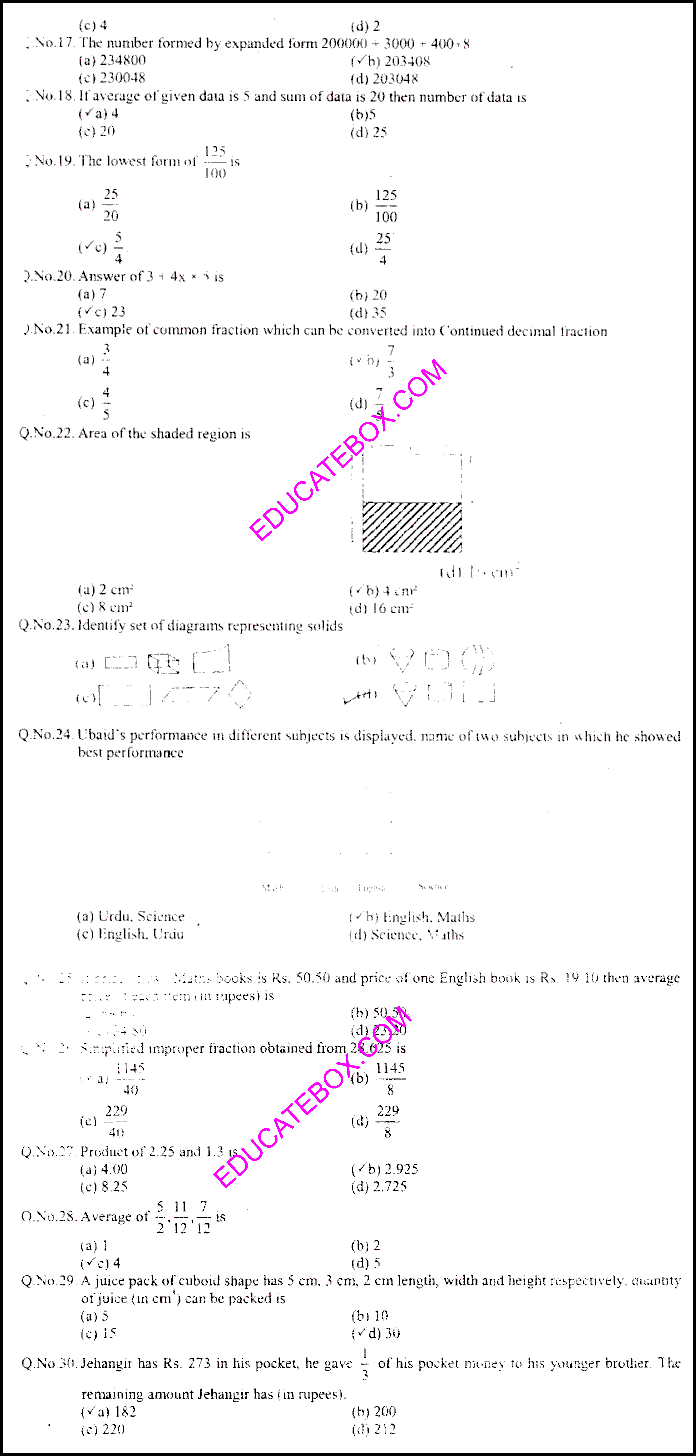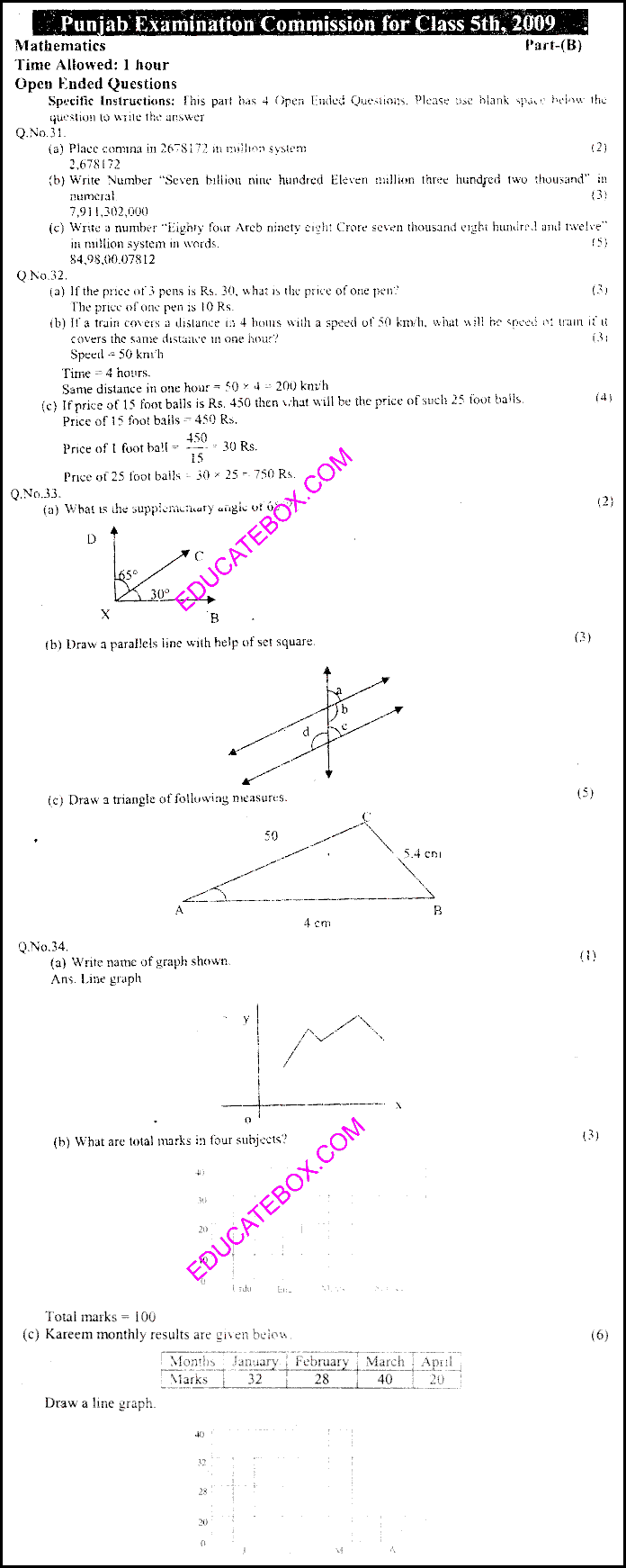# Past Paper Maths (EM) 5th Class 2009 Punjab Board (PEC) Solved Paper

0
745

Past Paper Maths (English Medium) 5th Class 2009 Punjab Board (PEC) Solved Paper

Class 5 Maths paper of 2009 consists of following multiple choice questions:

• How can we write numbers 813,138,831,318 in descending order?
• Shape of square
• What is approximate value of 4.236 up to 2 decimal place? The correct answer is 4.24.
• About set of equivalent fraction.
• What are x-axis and y-axis in a graph?
• Find Area of rectangle in a graph.
• How can we write decimal number 46 in Roman Number System?
• What is the greatest number formed by digits 1,5,6,2,7,0,8?
• What is lowest form of 3.25?
• What is addition of 1.024 and 3.370 up to 1 decimal place? The answer is 4.4.
• Find set of complementary angles in the diagram.
• Solution of 35.3211-13.83 correct up to 1 decimal places is 21.5.
• Find the set of parallel lines in the figure.
• If price of one chocolate bar is 2.25 then price of 4 chocolate bar is Rs. 9.
• Find the area of shaded region

Some of the objective questions are as follows:

• Place comma in 2678172 in million system
• Write number “Seven billion nine hundred Eleven million three hundred two thousand” in numeral
• Write a number “Eight four Arab ninety eight Crore seven thousand eight hundred and twelve” in million system in words.
• If the price of 3 pens is Rs. 30 what is the price of one pen?
• If a train covers a distance in 4 hours with a speed of 50 kmh. What will be speed of train if it covers the same distance in one hour?
• If price of 15 footballs is Rs. 450 then what will be the price of such 25 foot balls.
• What is the supplementary angle of 65
• Write name of graph shown.

Objective TypePast Paper Maths (English Medium) 5th Class 2009 Punjab Board (PEC) Solved Paper – Page 2Past Paper Maths (English Medium) 5th Class 2009 Punjab Board (PEC) Solved Paper – Page 3Past Paper Maths (English Medium) 5th Class 2009 Punjab Board (PEC) Solved Paper – Page 4

Subjective Type